# Quantitative Aptitude Quiz for SSC(Mix): 06 April 2021

Updated Tue, 06 Apr 2021 02:55 PM ISTSource: safalta
1. What is the digit at the hundredths place of the number N =?

### Free Demo Classes

a) 4
b) 5
c) 6
d) 7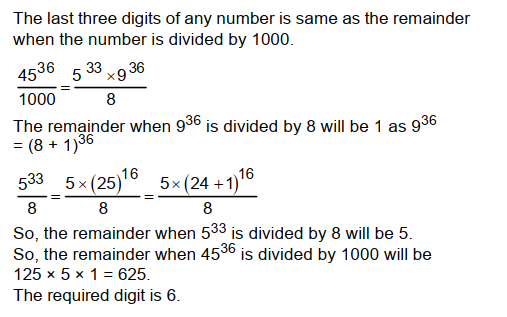2. After three successive changes (two increases and one decrease, not necessarily in that particular order), the price of a watch became (154/125) of its initial price. If the percentage changes are P, 2P and 4P respectively, then what is the value of 4P?

a) 20% or 40%
b) 40% or 200%
c) 200% or 8%
d) 8% or 20%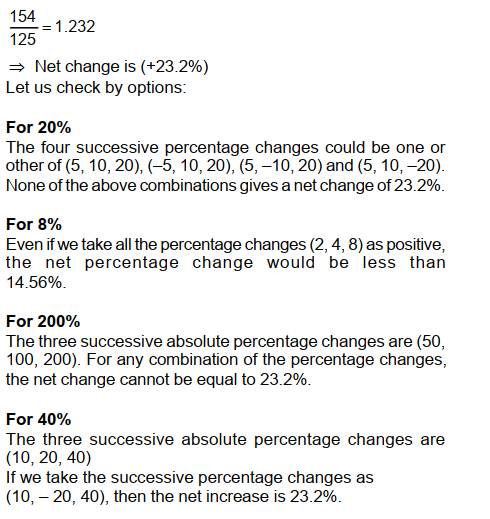3. The average weight of 47 balls is 10 gm. If the weight of the bag is included, the calculated average weight per ball increases by 0.3 gm. What is the weight of the bag?

a) 16.1 gm
b) 15.1 gm
c) 14.1 gm
d) 13.1 gm

Sum of weight of 47 balls = 10 × 47 = 470 gm

Average weight per ball increases by 0.3 gm

Weight of the bag = 10.3 × 47 - 10 × 47 = 14.1 gm

4. Which of the following divides 34^3 + 25^3 + 30^3 + 21^3 ?

I – 2

II – 5

III – 11

a)  only I
b)  only II
c)  only III
d)  I, II & III5.The difference of compound interest and simple interest for 3 years and for 2 years are in ratio 23 : 7 respectively. What is rate of interest per annum (in %)?

a) 100/7
b) 200/7
c) 50/7
d) 150/7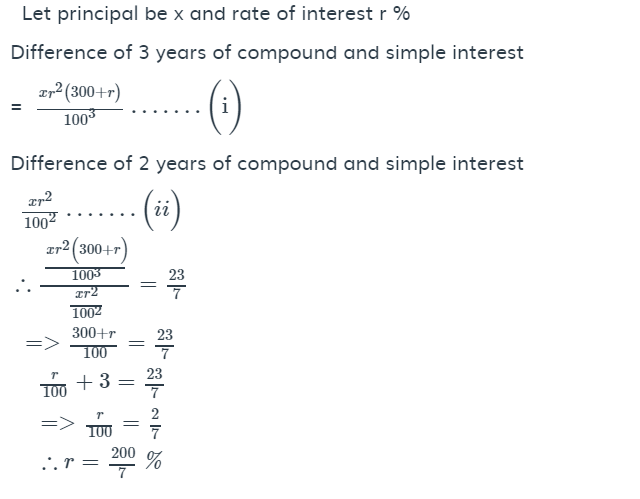6. In a five hour test, half way through, out of total Number of 300 questions Abhi answered 30% of the questions. What should be the percentage increase in average rate during the rest of the time so that Abhi can answer all the questions?

a)  33.33%
b)  166.67%
c)  133.33%
d)  66.67%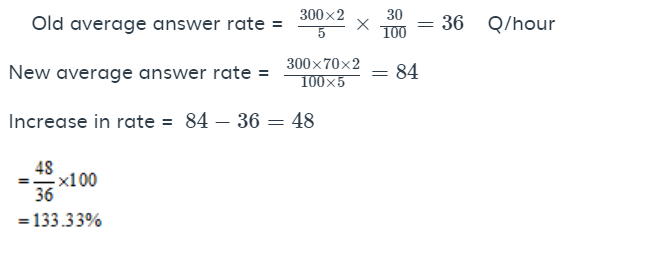7. Two metallic right circular cones having their heights 7.6 cm and 9.2 cm and the radii of their bases are 4.2 cm both are melted together and recast into a sphere. What is the radius of the sphere?

a) 4.2 cm
b) 4.9 cm
c) 3.5 cm
d) 5.6 cm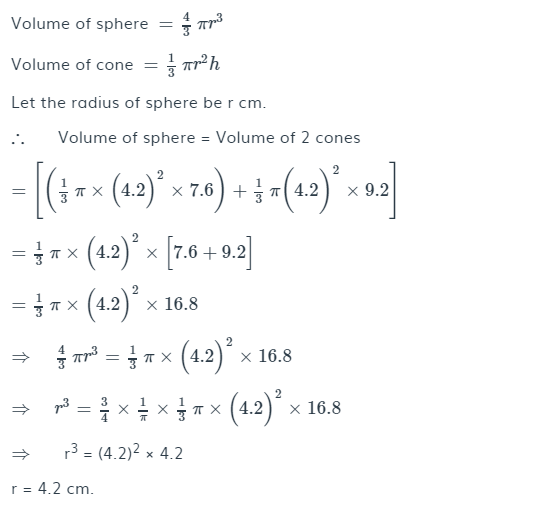8.  Rs. 5887 is divided between Shyam and Ram such that Shyam’s share at the end of 9 year is equal to Ram’s share at the end of 11 years, compounded annually at the rate of 5%. Find the share of Shyam.

a) 3087
b) 3187
c) 2987
d) 2887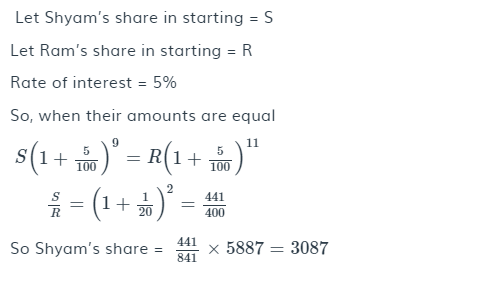9.  Cone is cut out from a hemisphere in such a way that the radius and the height of the cone are equal to the radius of the hemisphere. Find the ratio of the volume of the cone and the remaining volume of the hemisphere.

a) 1 : 2
b) 2 : 1
c) 1 : 1
d) 3 : 1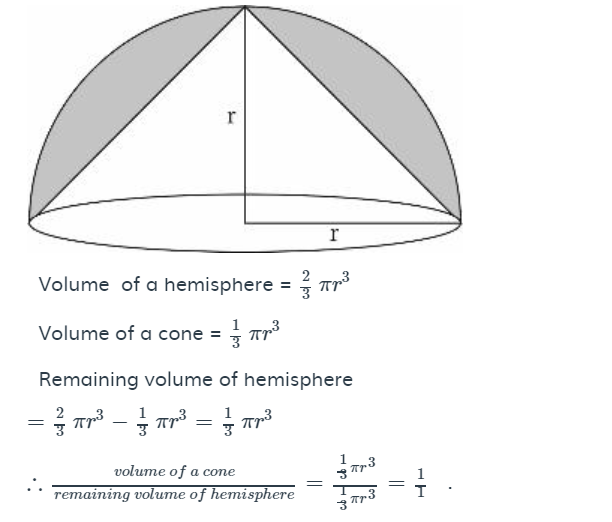10. Point P is the midpoint of segment AB. Co-ordinates of P are (3, 1) and B are (5, -4). What are the co-ordinates of point A?

a) (1, 6)
b) (1, -6)
c) (-1, 6)
d) (-1, -6)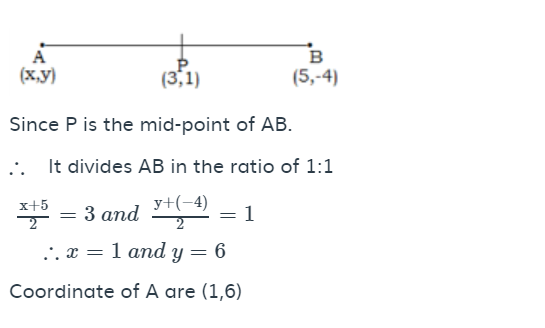### Disclaimer

अपनी वेबसाइट पर हम डाटा संग्रह टूल्स, जैसे की कुकीज के माध्यम से आपकी जानकारी एकत्र करते हैं ताकि आपको बेहतर अनुभव प्रदान कर सकें, वेबसाइट के ट्रैफिक का विश्लेषण कर सकें, कॉन्टेंट व्यक्तिगत तरीके से पेश कर सकें और हमारे पार्टनर्स, जैसे की Google, और सोशल मीडिया साइट्स, जैसे की Facebook, के साथ लक्षित विज्ञापन पेश करने के लिए उपयोग कर सकें। साथ ही, अगर आप साइन-अप करते हैं, तो हम आपका ईमेल पता, फोन नंबर और अन्य विवरण पूरी तरह सुरक्षित तरीके से स्टोर करते हैं। आप कुकीज नीति पृष्ठ से अपनी कुकीज हटा सकते है और रजिस्टर्ड यूजर अपने प्रोफाइल पेज से अपना व्यक्तिगत डाटा हटा या एक्सपोर्ट कर सकते हैं। हमारी Cookies Policy, Privacy Policy और Terms & Conditions के बारे में पढ़ें और अपनी सहमति देने के लिए Agree पर क्लिक करें।

Agree

Get free counselling session